# Answer to Question #4925 in Algebra for jojo alex

Question #4925
the sum of 2 numbers is 16 the sum of their reciprocals is 1/3 find the number ?
1
2011-11-01T11:24:35-0400
Solution
We have two unknown numbers and two conditions on these numbers.
Let&#039;s designate these numbers through x , y.
Let&#039;s make system of the equations.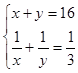Let&#039;s solve system of the equations.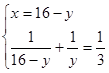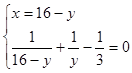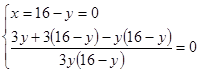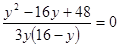=&gt;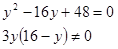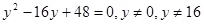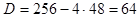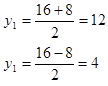Thus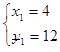or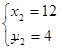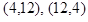Answer: These numbers are 4 and 12.

Need a fast expert's response?

Submit order

and get a quick answer at the best price

for any assignment or question with DETAILED EXPLANATIONS!

jayashree
01.11.11, 12:12

a+b=16
1/a+1/b=1/3
ab=48
a*(16-a)=48
a2-16a+48=0
a2-12a-4a+48=0
a(a-12)-4(a-12)=0
a=4,12

ihsan
31.10.11, 22:34

the numbers are following
1st number = 4
2nd number = 12